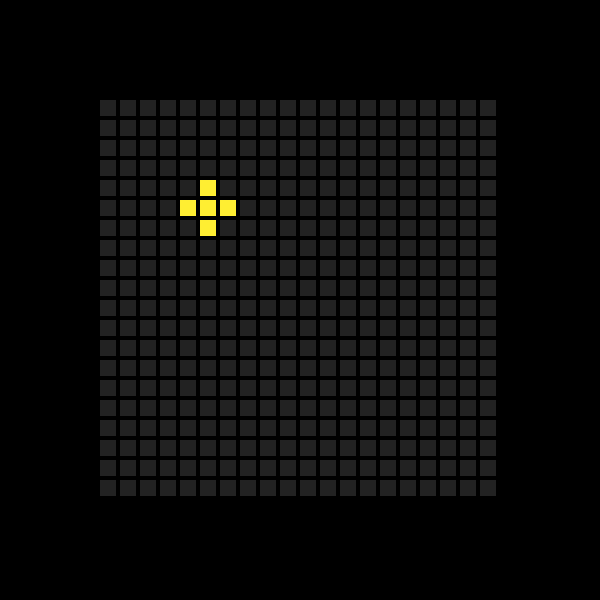sketch.js
1
2
3
4
5
6
7
8
9
10
11
12
13
14
15
16
17
18
19
20
21
22
23
24
25
26
27
28
29
30
31
32
33
34
35
36
37
38
39
40
41
42
43
44
45
46
``````// Constants
// Cell initialization

function setup() {
// Setup boilerplate

cells[cellIndex(5, 5)] = 1;
let neighbors = neighborCells(5, 5);
for (let i=0;i<neighbors.length;i++) {
cells[neighbors[i]] = 1;
}

renderCells();
}

function northIndex(x, y) {
if (y===0) { return -1; }
return cellIndex(x, y-1);
}

function southIndex(x, y) {
if (y===ROWS-1) { return -1; }
return cellIndex(x, y+1);
}

function eastIndex(x, y) {
if (x===COLUMNS-1) { return -1; }
return cellIndex(x+1, y);
}

function westIndex(x, y) {
if (x===0) { return -1; }
return cellIndex(x-1, y);
}

function neighborCells(x, y) {
let neighbors = [];
neighbors.push(northIndex(x, y));
neighbors.push(southIndex(x, y));
neighbors.push(eastIndex(x, y));
neighbors.push(westIndex(x, y));
return neighbors.filter(n => n !== -1);
}

// cellIndex()
// renderCells()``````
Preview
``````

``````Prevents attempting to return neighbor cells that are "off-grid".